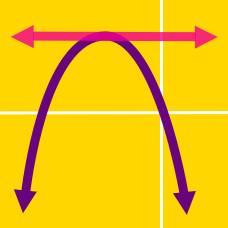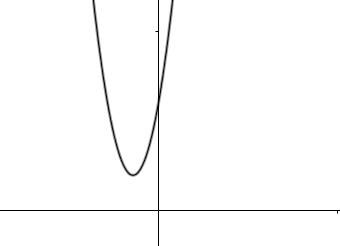Algebra

# Completing the Square - Find the VertexWhat is the minimum value of the quadratic function $y=2x^2+4x+16?$

The vertex of the parabola $y=x^2-12x+40$ is $P=(a, b)$. If the $y$-intercept is $Q=(0, c)$, what is the value of $a+b+c$?

If a parabola $y=ax^2-bx+c$ has its vertex at point $(5, -8)$ and passes through the point $(1, 8)$, what is the value of $a+b+c$?

For fixed real numbers $a$ and $b$, the parabola $y=2x^2-4ax+2a^2-b^2+16b$ has its vertex on parabola $y=x^2-8x+80$. What is the value of $a+b$?

Given the parabola $y=-x^2+8x+1,$ what is the maximum $y-$coordinate over all points on the parabola?

×